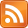(I'm just writing this to help myself remember. YMMV.)

This post is very indebted to Mateus Araújo, whose exposition of this subject is the best I've found for a non-physicist but mathy audience. Any mistakes are mine alone, because I am far from an expert on this topic and indeed don't even understand the mathematical framework of quantum mechanics.

## What is Bell's Theorem?

Bell's Theorem is how we know that quantum mechanics must be "weird"; regardless of what model we choose to explain these phenomena, that model must give up some fundamental characteristics of classical mechanics. Specifically, its content is mathematical and it is a "proof by contradiction" where the last step is done by experiment. (These are called Bell tests.) We end up having to discard one of our axioms.

You could imagine a world where we had classical mechanics, then Bell's Theorem experiments were done and forced us into quantum mechanics. This is not how it happened; we actually first had quantum mechanics, then Bell's Theorem (his paper On the Einstein Podolsky Rosen paradox in 1964), then experimental tests verifying it which got more and more loophole-free (last and strongest one in 2015).

The experiments are conceptually the same as the following situation: Alice and Bob are playing a game. They each receive a bit (0 or 1) simultaneously and must output another bit (0 or 1). They are physically separated in such a way that the speed-of-light limit prohibits interaction between them in the time between input and output.

Alice and Bob win and lose according to the following table.

Inputs Output same Output different
Alice: 0
Bob: 0
Win Lose
Alice: 0
Bob: 1
Win Lose
Alice: 1
Bob: 0
Win Lose
Alice: 1
Bob: 1
Lose Win

So, for example, if Alice and Bob receive 00 respectively and output 00 back, they win. If they output 01 back, they lose. But if they receive 11 and they output 01, they would win. They are allowed to communicate before the game starts, but not after it begins.

Common sense indicates (and mathematics backs up) that the best strategy in this contrived game, given that you can't communicate, is to agree ahead of time to always both output the same bit (say 0). You'll win 75% = $\frac{3}{4}$ of the time.

Yet, in the world we live in, if we set up Alice and Bob in certain ways (of course in the experiments they are not really humans, but machines), they can win $\frac{2 + \sqrt{2}}{4}$ $\approx$ 85% of the time. Quantum mechanics would say that these are situations where they have shared an entangled state before the game starts. Then, by taking actions on their entangled state, they can in a probabilistic way share information that allows them to win more often. (See here for a full explanation I don't yet understand.)

Bell's Theorem is the formalization of the idea that, under models that have the same fundamental characteristics as classical mechanics, this situation is impossible. Hence, such models do not describe the world we live in.

## Okay. What are the "fundamental characteristics"?

Technically there are two, and you can pick which one you want to lose.

#### Local Causality

This is much more discussed and commonly though of as being "given up". This is the idea that "causes are close to their effects", or, in relativistic terms, events in a spacetime region $A$ depend only on the contents of its past light cone $\Lambda$, and not on the contents of some disconnected region $B$. (Not sure if this is the same as Local Realism.)(image credit to Mateus Araújo)

This is one way understanding of the concept "no action at a distance" which is central to our intuitive conceptions of physics. (Note that, in Mateus's blog post, "no action at a distance" is a specific condition, weaker than this.)

Losing this is intense -- some idea of "locality" is suprisingly central to our understanding of the world. If the outcomes of our actions on Earth could depend on the state of some molecule on Alpha Centauri, how can we do science? Yet in practice, we seem to be muddling along.

#### No conspiracy

To see this objection, observe that the universe can be perfectly classical (billiard balls colliding in a void) and Alice and Bob still win their games if they are simply fated to do so. That is, if the initial conditions of the universe are set up such that Alice and Bob win 85 out of the 100 games they play, then their settings will be chosen such that they do so, probability be damned.

We need to posit that the universe is not specifically designed to frustrate us -- equivalently, that we as the operators of the machines which are Alice and Bob are choosing their settings in a way uncorrelated with the rest of the experiment, as we think we are*. Some (me) may find this even worse to give up than the previous condition. Elsewhere this is called Superdeterminism.

## Does Bell's Theorem imply that we live in a nondeterministic universe?

No. For example, Bohmian mechanics is a model which is deterministic, though it gives up local causality (and indeed more).

There are a variety of interpretations of quantum mechanics, but the most mainstream ones are indeed nondeterministic.

* In experiments, amusing lengths are gone to to protect against this. For example, from this paper: "Finally, Alice and Bob each have a different predetermined pseudorandom source that is composed of various popular culture movies and TV shows, as well as the digits of π, XORed together."